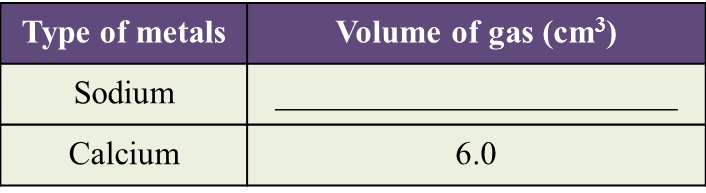Question 1:
Diagram 1.1 and Diagram 1.2 show an experiment to study the reactivity of metals with water.(a) Observe Diagram 1.1. State the volume of gas collected.(b) Based on the result in Table above, state one inference. [1 mark]

(c) State the variables in this experiment.
(i) Constant variable [1 mark]
(ii) Responding variable [1 mark]

(d) Based on this experiment, mark (\/) the metal which is more reactive. [1 mark]